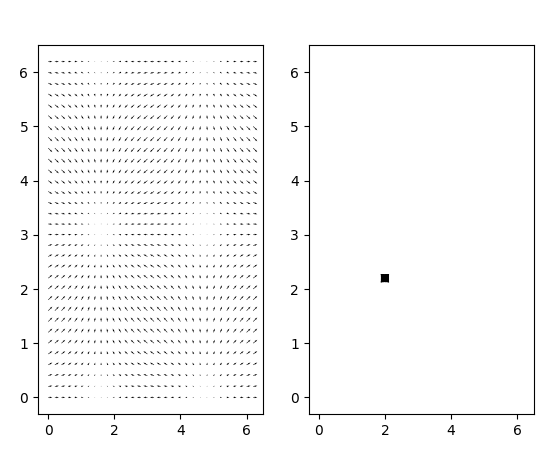# Why can't I use `set_offsets` on quiver?

The quiver docs claim that the only api method is `set_UVC` and that the positions are fixed. And messing around that certainly seems to be true. Does anyone know what goes wrong when I use set_offsets on a quiver object? and in particular if there is something I could do to animate the XY positions, or something to change in the codebase to allow that.

``````import numpy as np
import matplotlib.pyplot as plt
fig, axs = plt.subplots(1,2)

X, Y = np.meshgrid(np.arange(0, 2 * np.pi, .2), np.arange(0, 2 * np.pi, .2))
U = np.cos(X)
V = np.sin(Y)

axs.quiver(X, Y, U, V, units='width')
Q = axs.quiver(X, Y, U, V, units='width')

# update coords
X, Y = np.meshgrid(np.arange(0, 2 * np.pi, .2)+2, np.arange(0, 2 * np.pi, .2))
U = np.cos(X)
V = np.sin(Y)

# setting all the things I can think of
Q.X = X
Q.Y = Y
Q.XY = np.column_stack([X, Y])
Q.set_offsets(Q.XY)
Q.set_UVC(U=U, V=V)

plt.show()

``````

gives:oh interesting. Even if I don’t modify the XY array then `set_offsets` still destroys the plot.

ahhh because you need to ravel X and Y.